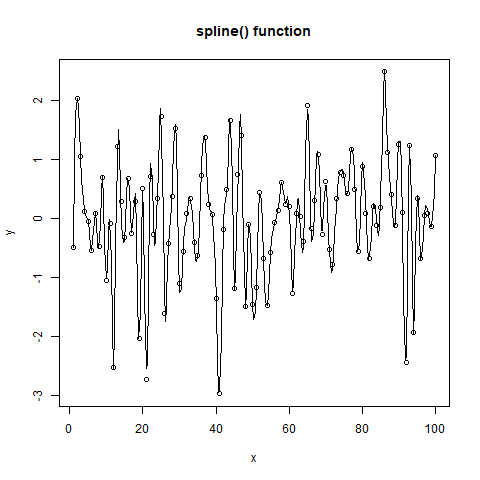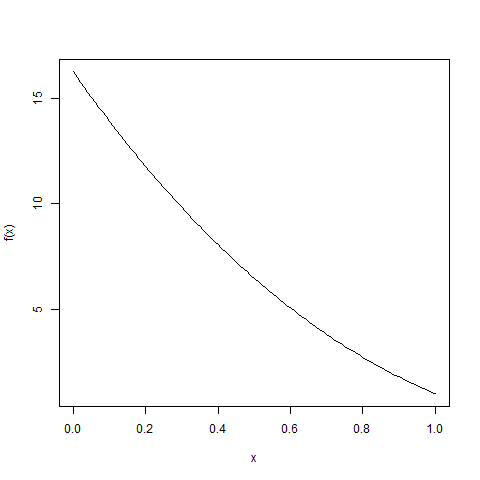# Get a List of points obtained by Interpolation in R Programming – spline() and splinefun() Function

In R programming, `spline()` and `splinefun()` function is used to create a list of points obtained by interpolation. It performs cubic spline interpolation of given data points.

Syntax:
spline(x, y, method)
and
splinefun(x, y, method)

Parameters:
x, y: represents vectors giving the points for interpolation
method: represents the type of spline interpolation to be used

To know about more optional parameters of both the functions, use below command in console:

```help("spline")
```

Example 1:

 `# Coordinates ` `n <- 100 ` `x <- 1:n ` `y <- ``rnorm``(n) ` ` `  `# Output to be present as PNG file ` `png``(file = ``"splineGFG.png"``) ` ` `  `# Spline() function ` `plot``(x, y, main = ``"spline() function"``) ` `lines``(``spline``(x, y)) ` ` `  `# Saving the file ` `dev.off``() `

Output:Example 2:

 `# Coordinates ` `n <- 100 ` `x <- 1:n ` `y <- ``sin``((x-0.5)*``pi``) ` ` `  `# Output to be present as PNG file ` `png``(file = ``"splinefunGFG.png"``) ` ` `  `f <- ``splinefun``(x, y) ` `curve``(``f``(x)) ` ` `  `# Saving the file ` `dev.off``() `

Output:My Personal Notes arrow_drop_upBlockchain Enthusiast

If you like GeeksforGeeks and would like to contribute, you can also write an article using contribute.geeksforgeeks.org or mail your article to contribute@geeksforgeeks.org. See your article appearing on the GeeksforGeeks main page and help other Geeks.

Please Improve this article if you find anything incorrect by clicking on the "Improve Article" button below.

Article Tags :

Be the First to upvote.

Please write to us at contribute@geeksforgeeks.org to report any issue with the above content.